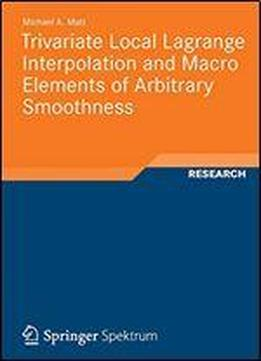Trivariate Local Lagrange Interpolation And Macro Elements Of Arbitrary Smoothness by Michael Andreas Matt / 2012 / English / PDF

Michael A. Matt constructs two trivariate local Lagrange interpolation methods which yield optimal approximation order and Cr macro-elements based on the Alfeld and the Worsey-Farin split of a tetrahedral partition. The first interpolation method is based on cubic C1 splines over type-4 cube partitions, for which numerical tests are given. The second is the first trivariate Lagrange interpolation method using C2 splines. It is based on arbitrary tetrahedral partitions using splines of degree nine. The author constructs trivariate macro-elements based on the Alfeld split, where each tetrahedron is divided into four subtetrahedra, and the Worsey-Farin split, where each tetrahedron is divided into twelve subtetrahedra, of a tetrahedral partition. In order to obtain the macro-elements based on the Worsey-Farin split minimal determining sets for Cr macro-elements are constructed over the Clough-Tocher split of a triangle, which are more variable than those in the literature.

views: 101